## What the Maximum Current the Thick Film Ceramic PCB can be Carried?

A lot of customers ask us what the biggest current his circuit can be carried, or how can I got a 6Â Amps trace? We believe most of engineer design the thick film ceramic PCB, he will consider that, so we share information bellowing and hope itâ€™ll be helpful for you.

When you try to know current, you also need to know the watts of trace will be, and then need to know the power density of thick film ceramic PCBÂ firstly. The power density for conductor itself should be limited to max 600 Watt/inch2 of conductor surface. And power density for an Al2O3 substrate should be limited to 8 Watts/in2 (for the total of all conductors on top of it).

If you have a Ag conductor line of 0.3inch long, 0.010 inch wide (=30 square (0.3/0.01)), the surface area is 0.3 x 0.01 = 0.003 in2. This means the carrying powder P is limited to 600 (power density) x 0.003 (area) = 1.8 watts.

Assume conductor resistivity is 6mOhm/sq/12.5um (material P/N: ESL9562), the resistance value R = 30 sq x 6mOhm = 0.18 ohm.

Power = I2 x R, or I2 =Power/R=1.8/0.18 = 10, so the carrying current limit I = 3.16 amps. Or in a short equation,

I (amps) = line width (inch) x (power density)1/2 / (sheet resistance, ohm) 1/2

1. If the trace keep 0.3 inch long, but change width to 0.02inch, (=15 square (0.3/0.02), the surface area is 0.3 x 0.02 = 0.006 in2. This means the carrying powder P is limited to 600 (power density) x 0.006 (area) = 6 watts.
And resistance value for that trace will be 15 square x 6mOhm = 0.09 ohm, and then 3.6/0.09= 40, I=6.325 amps.
2. If the trace width keep 0.01inch, but change line to 0.6 inch long (=60 square (0.6/0.01), the surface area is 0.6 x 0.01 = 0.006 in2. This means the carrying powder P is the same as 6 watts. (600×0.006).
But the resistance value for that trace will change to be 60 square x 6mOhm = 0.36 ohm, and then 3.6/0.36= 10, I=3.16 amps. So you can see, itâ€™s no useful to change the trace line.

So if you want to have bigger current for thick film ceramic PCB, then you need to increase the width of trace.

Hope above is clear for everybody. Please leave your comments on that power density ofÂ thick film ceramic PCB.

#### You may also like

•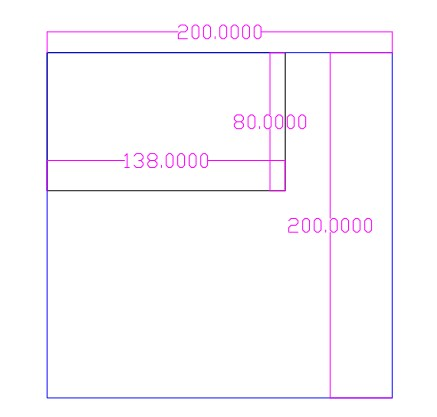•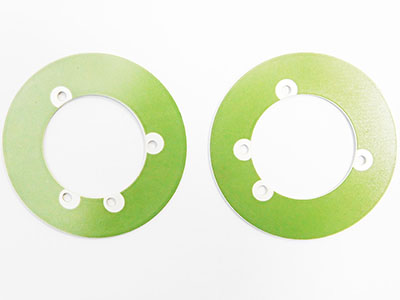•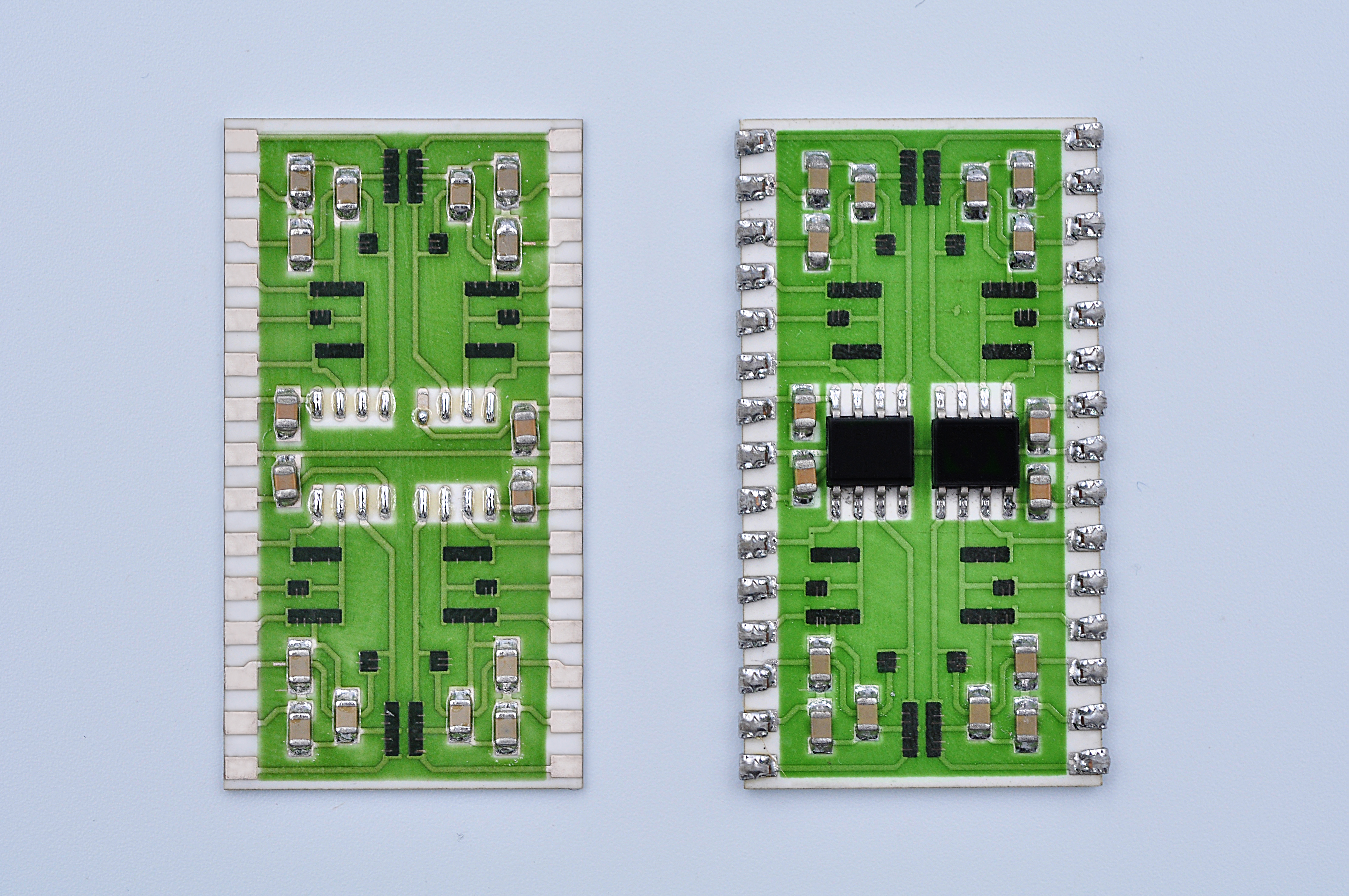••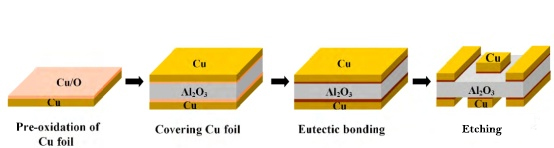•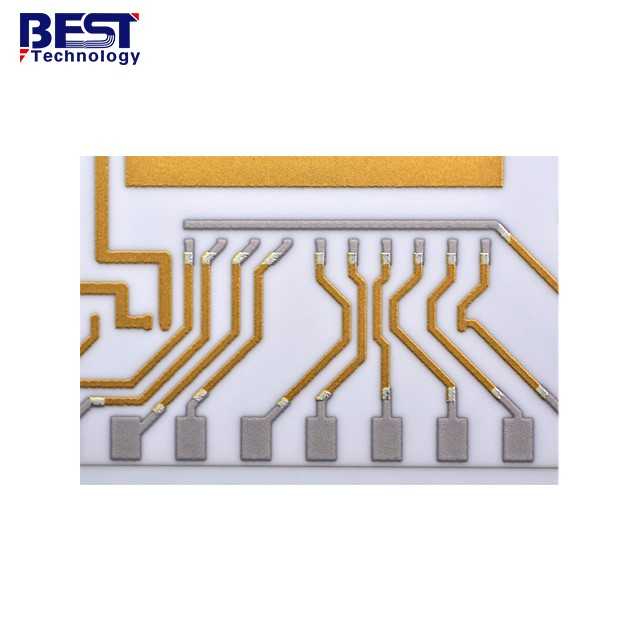•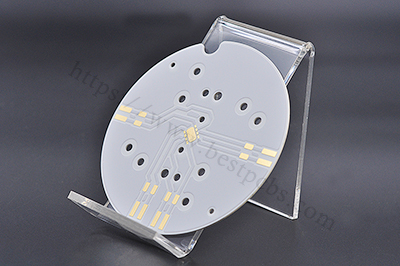•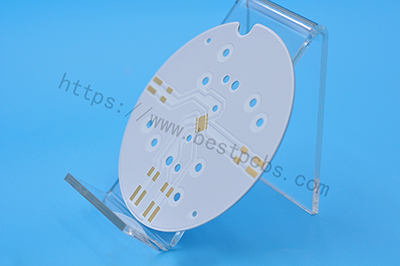Tags: ,

### One Response to “What the Maximum Current the Thick Film Ceramic PCB can be Carried?”

1. Portiva healthcare virtual assistant jobs

blog topic